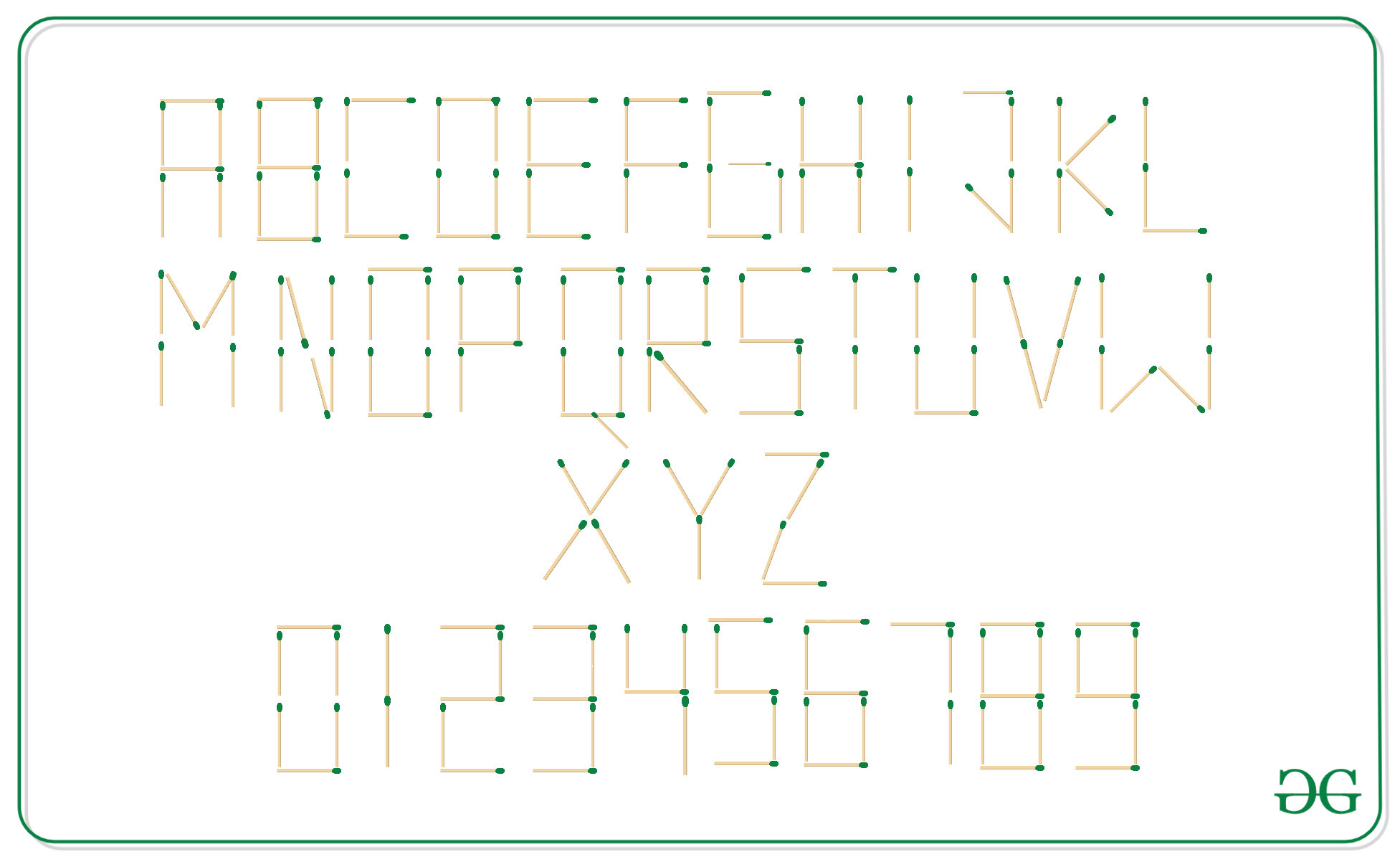# Sort the strings based on the numbers of matchsticks required to represent them

Given an array arr[] of N strings, the task is to sort these strings according to the numbers of sticks required to represent them.

Examples:

Input: arr[] = { “123”, “ABC”, “88” }
Output: 123 88 ABC
Explanation:
Sticks Required by each strings are as follows:
123 -> 12 sticks
88 -> 14 sticks
ABC -> 17 sticks

Input: arr[] = { “GEEKS”, “FOR”, “GEEKSFORGEEKS” }
Output: FOR GEEKS GEEKSFORGEEKS
Explanation:
Sticks required by each strings are as follows:
FOR => 16 sticks
GEEKS => 25 sticks
GEEKSFORGEEKS => 66 sticks

Approach: The idea is to compute the number of sticks required by each string with the help of the counts of the sticks required for each character. Then, store the count of the sticks and the strings into an array of pairs. Finally, sort them according to the number of sticks required.

Below figure is shown for the count of sticks required to represent each character:Below is the implementation of the above approach:

## C++

 `// C++ implementation to sort the ` `// strings with the number of ` `// sticks required to represent them ` ` `  `#include ` ` `  `using` `namespace` `std; ` ` `  `// Stick[] stores the count ` `// of sticks required to ` `// represent the alphabets ` `int` `sticks[] = { 6, 7, 4, 6, 5, 4, 6, ` `                 ``5, 2, 4, 4, 3, 6, 6, ` `                 ``6, 5, 7, 6, 5, 3, 5, ` `                 ``4, 6, 4, 3, 4 }; ` ` `  `// Number[] stores the count ` `// of sticks required to ` `// represent the numerals ` `int` `number[] = { 6, 2, 5, 5, 4, 5, 6, ` `                 ``3, 7, 6 }; ` ` `  `// Function that return the count of ` `// sticks required to represent ` `// the given string str ` `int` `countSticks(string str) ` `{ ` `    ``int` `cnt = 0; ` ` `  `    ``// Loop to iterate over every ` `    ``// character of the string ` `    ``for` `(``int` `i = 0; str[i]; i++) { ` ` `  `        ``char` `ch = str[i]; ` ` `  `        ``// Add the count of sticks ` `        ``// required to represent the ` `        ``// current character ` `        ``if` `(ch >= ``'A'` `&& ch <= ``'Z'``) { ` `            ``cnt += sticks[ch - ``'A'``]; ` `        ``} ` `        ``else` `{ ` `            ``cnt += number[ch - ``'0'``]; ` `        ``} ` `    ``} ` `    ``return` `cnt; ` `} ` `// Function to sort the array ` `// according to the number of ` `// sticks required to represent it ` `void` `sortArr(string arr[], ``int` `n) ` `{ ` `    ``// Vector to store the number ` `    ``// of sticks required with ` `    ``// respective strings ` `    ``vector > vp; ` ` `  `    ``// Inserting number of sticks ` `    ``// with respective strings ` `    ``for` `(``int` `i = 0; i < n; i++) { ` `        ``vp.push_back( ` `            ``make_pair(countSticks(arr[i]), ` `                      ``arr[i])); ` `    ``} ` ` `  `    ``// Sort the vector, ` `    ``sort(vp.begin(), vp.end()); ` ` `  `    ``// Print the sorted vector ` `    ``for` `(``int` `i = 0; i < vp.size(); i++) ` `        ``cout << vp[i].second << ``" "``; ` `} ` ` `  `// Driver Code ` `int` `main() ` `{ ` `    ``string arr[] = { ``"GEEKS"``, ``"FOR"``, ` `                     ``"GEEKSFORGEEKS"` `}; ` `    ``int` `n = ``sizeof``(arr) / ``sizeof``(arr); ` ` `  `    ``sortArr(arr, n); ` ` `  `    ``return` `0; ` `} `

Output:

```FOR GEEKS GEEKSFORGEEKS
```

Time Complexity: O(N * log N)

Don’t stop now and take your learning to the next level. Learn all the important concepts of Data Structures and Algorithms with the help of the most trusted course: DSA Self Paced. Become industry ready at a student-friendly price.

My Personal Notes arrow_drop_upCheck out this Author's contributed articles.

If you like GeeksforGeeks and would like to contribute, you can also write an article using contribute.geeksforgeeks.org or mail your article to contribute@geeksforgeeks.org. See your article appearing on the GeeksforGeeks main page and help other Geeks.

Please Improve this article if you find anything incorrect by clicking on the "Improve Article" button below.